Illustration of Model Identification: Spleen Data

When your model involves measurement errors in variables and you need to use latent true scores in the regression or structural equation, you might encounter some model identification problems in estimation if you do not put certain identification constraints in the model. An example is shown in the section Regression with Measurement Errors in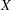and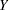for the corn data. You "solved" the problem by assuming a deterministic model with perfect prediction in the structural model. However, this assumption could be very risky and does not lead to estimation results that are substantively different from the model with measurement error only in.

This section shows how you can apply another set of constraints to make the measurement model with errors in bothandidentified without assuming the deterministic structural model. First, the identification problem is illustrated here again in light of the PROC CALIS diagnostics.

The following example is inspired by Fuller (1987, pp. 40–41). The hypothetical data are counts of two types of cells in spleen samples: cells that form rosettes and nucleated cells. It is reasonable to assume that counts have a Poisson distribution; hence, the square roots of the counts should have a constant error variance of 0.25. You can use PROC CALIS to fit this regression model with measurement errors inandto the data. (See the section Regression with Measurement Errors inandfor model definitions.) However, before fitting this target model, it is illustrative to see what would happen if you do not assume the constant error variance.

The following statements show the LINEQS specification of an errors-in-variables regression model for the square roots of the counts without constraints on the parameters:

```data spleen;
input rosette nucleate;
sqrtrose=sqrt(rosette);
sqrtnucl=sqrt(nucleate);
datalines;
4 62
5 87
5 117
6 142
8 212
9 120
12 254
13 179
15 125
19 182
28 301
51 357
;
```
```proc calis data=spleen;
lineqs factrose = beta * factnucl + disturb,
sqrtrose =        factrose + err_rose,
sqrtnucl =        factnucl + err_nucl;
variance
factnucl = v_factnucl,
disturb  = v_disturb,
err_rose = v_rose,
err_nucl = v_nucl;
run;
```

This model is underidentified. You have five parameters to estimate in the model, but the number of distinct covariance elements is only three.

In the LINEQS statement, you specify the structural equation and then two measurement equations. In the structural equation, the variables factrose and factnucl are latent true scores for the corresponding measurements in sqrtrose and sqrtnucl, respectively. The structural equation represents the true variable relationship of interest. You name the regression coefficient parameter as beta and the error term as disturb in the structural model. (For structural equations, you can use names with prefix 'D' or 'd' to denote error terms.) The variance of factnucl and the variance of disturb are also parameters in the model. You name these variance parameters as v_factnucl and v_disturb in the VARIANCE statement. Therefore, you have three parameters in the structural equation.

In the measurement equations, the observed variables sqrtrose and sqrtnucl are specified as the sums of their corresponding true latent scores and error terms, respectively. The error variances are also parameters in the model. You name them as v_rose and v_nucl in the VARIANCE statement. Now, together with the three parameters in the structural equation, you have a total of five parameters in your model.

All variance specifications in the VARIANCE statement are actually optional in PROC CALIS. They are free parameters by default. In this example, it is useful to name these parameters so that explicit references to these parameters can be made in the following discussion.

PROC CALIS displays the following warning when you fit this underidentified model:

```   WARNING: Estimation problem not identified: More parameters to
estimate ( 5 ) than the total number of mean and
covariance elements ( 3 ).
```

In this warning, the three covariance elements refer to the sample variances of sqrtrose and sqrtnucl and their covariance. PROC CALIS diagnoses the parameter indeterminacy as follows:

```   NOTE: Covariance matrix for the estimates is not full rank.
NOTE: The variance of some parameter estimates is zero or
some parameter estimates are linearly related to other
parameter estimates as shown in the following equations:

v_rose       =      -0.147856   +       0.447307   *   v_disturb

v_nucl       =    -110.923690   -       0.374367   *   beta       +
10.353896   *   v_factnucl     +   1.536613   *   v_disturb
```

With the warning and the notes, you are now certain that the model is underidentified and you cannot interpret your parameter estimates meaningfully.

Now, to make the model identified, you set the error variances to 0.25 in the VARIANCE statement, as shown in the following specification:

```proc calis data=spleen residual;
lineqs factrose = beta * factnucl + disturb,
sqrtrose =        factrose + err_rose,
sqrtnucl =        factnucl + err_nucl;
variance
factnucl = v_factnucl,
disturb  = v_disturb,
err_rose = 0.25,
err_nucl = 0.25;
run;
```

In the specification, you use the RESIDUAL option in the PROC CALIS statement to request the residual analysis. An annotated fit summary is shown in Figure 17.7.

Figure 17.7 Spleen Data: Annotated Fit Summary for the Just-Identified Model
Fit Summary
Chi-Square 0.0000
Chi-Square DF 0
Pr > Chi-Square .

You notice that the model fit chi-square is 0 and the corresponding degrees of freedom is also 0. This indicates that your model is "just" identified, or your model is saturated—you have three distinct elements in the sample covariance matrix for the estimation of three parameters in the model. In the PROC CALIS results, you no longer see the warning message about underidentification or any notes about linear dependence in parameters.

For just-identified or saturated models like the current case, you expect to get zero residuals in the covariance matrix, as shown in Figure 17.8:

Figure 17.8 Spleen Data: Residuals for the Just-identified Model
Raw Residual Matrix
sqrtrose sqrtnucl
sqrtrose 0.00000 0.00000
sqrtnucl 0.00000 0.00000

Residuals are the differences between the fitted covariance matrix and the sample covariance matrix. When the residuals are all zero, the fitted covariance matrix matches the sample covariance matrix perfectly (the parameter estimates reproduce the sample covariance matrix exactly).

You can now interpret the estimation results of this just-identified model, as shown in Figure 17.9:

Figure 17.9 Spleen Data: Parameter Estimated for the Just-Identified Model
Linear Equations
factrose =   0.3907 * factnucl + 1.0000   disturb
Std Err     0.0771   beta
t Value     5.0692
sqrtrose =   1.0000   factrose + 1.0000   err_rose
sqrtnucl =   1.0000   factnucl + 1.0000   err_nucl

Estimates for Variances of Exogenous Variables
Variable
Type
Variable Parameter Estimate Standard
Error
t Value
Latent factnucl v_factnucl 10.50458 4.58577 2.29069
Disturbance disturb v_disturb 0.38153 0.28556 1.33607
Error err_rose   0.25000
err_nucl   0.25000

Notice that because the error variance parameters for variables err_rose and err_nucl are fixed constants in the model, there are no standard error estimates for them in Figure 17.9. For the current application, the estimation results of the just-identified model are those you would interpret and report. However, to completely illustrate model identification, an additional constraint is imposed to show an overidentified model. In the section Regression with Measurement Errors inand, you impose a zero-variance constraint on the disturbance variable Dfy for the model identification. Would this constraint be necessary here for the spleen data too? The answer is no because with the two constraints on the variances of err_rose and err_nucl, the model has already been meaningfully specified and identified. Adding more constraints such as a zero variance for disturb would make the model overidentified unnecessarily. The following statements show the specification of such an overidentified model for the spleen data:

```proc calis data=spleen residual;
lineqs factrose = beta * factnucl + disturb,
sqrtrose =        factrose + err_rose,
sqrtnucl =        factnucl + err_nucl;
variance
factnucl = v_factnucl,
disturb  = 0.,
err_rose = 0.25,
err_nucl = 0.25;
run;
```

An annotated fit summary table for the overidentified model is shown in Figure 17.10.

Figure 17.10 Spleen Data: Annotated Fit Summary for the Overidentified Model
Fit Summary
Chi-Square 5.2522
Chi-Square DF 1
Pr > Chi-Square 0.0219
Standardized RMSR (SRMSR) 0.0745
RMSEA Estimate 0.6217
Bentler Comparative Fit Index 0.6535

The chi-square is 5.2522 (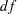=1,=0.0219). Overall, the model does not provide a good fit. The sample size is so small that the-value of the chi-square test should not be taken to be accurate, but to get a small-value with such a small sample indicates that it is possible that the model is seriously deficient.

This same conclusion can be drawn by looking at other fit indices in the table. In Figure 17.10, several fit indices are computed for the model. For example, the standardized root mean square residual (SRMSR) is 0.0745 and the adjusted goodness of fit (AGFI) is 0.1821. By conventions, a good model should have an SRMSR smaller than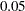and an AGFI larger than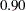. The root mean square error of approximation (RMSEA) (Steiger and Lind; 1980) is 0.6217, but an RMSEA belowis recommended for a good model fit (Browne and Cudeck; 1993). The comparative fit index (CFI) is 0.6535, which is also low as compared to the acceptable level at 0.90.

When you fit an overidentified model, usually you do not find estimates that match the sample covariance matrix exactly. The discrepancies between the fitted covariance matrix and the sample covariance matrix are shown as residuals in the covariance matrix, as shown in Figure 17.11.

Figure 17.11 Spleen Data: Residuals for the Overidentified Model
Raw Residual Matrix
sqrtrose sqrtnucl
sqrtrose 0.28345 -0.11434
sqrtnucl -0.11434 0.04613

As you can see in Figure 17.11, the residuals are nonzero. This indicates that the parameter estimates do not reproduce the sample covariance matrix exactly. For overidentified models, nonzero residuals would be the norm rather than exception, but the general goal is to find the "best" set of estimates so that the residuals are as small as possible.

The parameter estimates are shown in Figure 17.12.

Figure 17.12 Spleen Data: Parameter Estimated for the Overidentified Model
Linear Equations
factrose =   0.4034 * factnucl + 1.0000   disturb
Std Err     0.0508   beta
t Value     7.9439
sqrtrose =   1.0000   factrose + 1.0000   err_rose
sqrtnucl =   1.0000   factnucl + 1.0000   err_nucl

Estimates for Variances of Exogenous Variables
Variable
Type
Variable Parameter Estimate Standard
Error
t Value
Latent factnucl v_factnucl 10.45846 4.56608 2.29047
Disturbance disturb   0
Error err_rose   0.25000
err_nucl   0.25000

The estimate of beta in this model is 0.4034. Given that the model fit is bad and the zero variance for the error term disturb is unreasonable, beta could have been overestimated in the current overidentified model, as compared with the just-identified model, where the estimate of beta is only 0.3907. In summary, both the fit summary and the estimation results indicate that the zero variance for disturb in the overidentified model for the spleen data has been imposed unreasonably.

The purpose of the current illustration is not that you should not consider an overidentified model for your data in general. Quite the opposite, in practical structural equation modeling it is usually the overidentified models that are of the paramount interest. You can test or gauge the model fit of overidentified models. Good overidentified models enable you to establish scientific theories that are precise and general. However, most fit indices are not meaningful when applied to just-identified saturated models. Also, even though you always get zero residuals for just-identified saturated models, those models usually are not precise enough to be a scientific theory.

The overidentified model for the spleen data highlights the importance of setting meaningful identification constraints. Whether your resulting model is just-identified or overidentified, it is recommended that you do the following:

• Give priorities to those identification constraints that are derived from prior studies, substantive grounds, or mathematical basis.

• Avoid making unnecessary identification constraints that might bias your model estimation.